## SineLet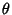be an Angle measured counterclockwise from the x-Axis along the arc of the Unit Circle. Then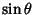is the vertical coordinate of the arc endpoint. As a result of this definition, the sine function is periodic with period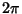. By the Pythagorean Theorem,also obeys the identity(1)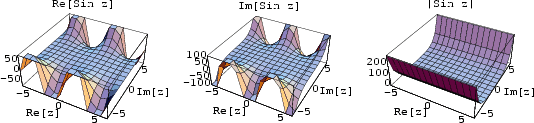The sine function can be defined algebraically by the infinite sum(2)

and Infinite Product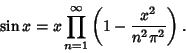(3)

It is also given by the Imaginary Part of the complex exponential(4)

The multiplicative inverse of the sine function is the Cosecant, defined as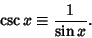(5)

Using the results from the Exponential Sum Formulas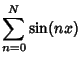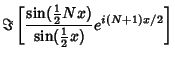(6)

Similarly,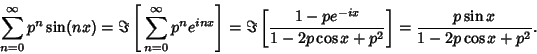(7)

Other identities include(8)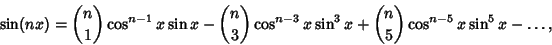(9)

whereis a Binomial Coefficient.

Cvijovic and Klinowski (1995) show that the sum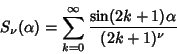(10)

has closed form for,(11)

whereis an Euler Polynomial.

A Continued Fraction representation of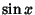is(12)

The value of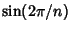is Irrational for allexcept 4 and 12, for whichand.

The Fourier Transform of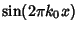is given by(13)

Definite integrals involvinginclude(14)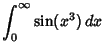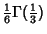(15)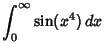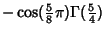(16)(17)

whereis the Gamma Function.

See also Andrew's Sine, Cosecant, Cosine, Fourier Transform--Sine, Hyperbolic Sine, Sinc Function, Tangent, Trigonometry

References

Abramowitz, M. and Stegun, C. A. (Eds.). Circular Functions.'' §4.3 in Handbook of Mathematical Functions with Formulas, Graphs, and Mathematical Tables, 9th printing. New York: Dover, pp. 71-79, 1972.

Cvijovic, D. and Klinowski, J. Closed-Form Summation of Some Trigonometric Series.'' Math. Comput. 64, 205-210, 1995.

Hansen, E. R. A Table of Series and Products. Englewood Cliffs, NJ: Prentice-Hall, 1975.

Project Mathematics! Sines and Cosines, Parts I-III. Videotapes (28, 30, and 30 minutes). California Institute of Technology. Available from the Math. Assoc. Amer.

Spanier, J. and Oldham, K. B. The Sine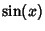and Cosine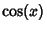Functions.'' Ch. 32 in An Atlas of Functions. Washington, DC: Hemisphere, pp. 295-310, 1987.